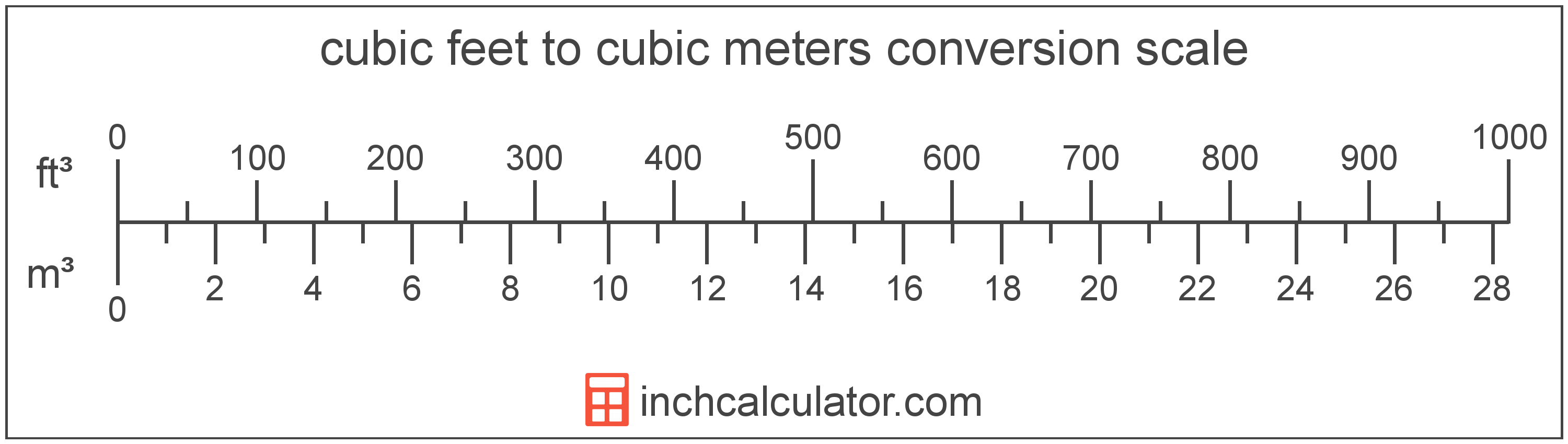# Cubic Meters to Cubic Feet Conversion

Enter the volume in cubic meters below to get the value converted to cubic feet.

(find cubic meters)
Results in Cubic Feet:1 m³ = 35.314667 ft³

## How to Convert Cubic Meters to Cubic FeetTo convert a cubic meter measurement to a cubic foot measurement, multiply the volume by the conversion ratio.

Since one cubic meter is equal to 35.314667 cubic feet, you can use this simple formula to convert:

cubic feet = cubic meters × 35.314667

The volume in cubic feet is equal to the cubic meters multiplied by 35.314667.

For example, here's how to convert 5 cubic meters to cubic feet using the formula above.
5 m³ = (5 × 35.314667) = 176.573337 ft³

## Cubic Meters

One cubic meter is equal to the volume of a cube with each edge measuring one meter.

The cubic meter, or cubic metre, is the SI derived unit for volume in the metric system. Cubic meters can be abbreviated as , and are also sometimes abbreviated as cu m, CBM, cbm, or MTQ. For example, 1 cubic meter can be written as 1 m³, 1 cu m, 1 CBM, 1 cbm, or 1 MTQ.

Try our cubic meters calculator to calculate the volume of a space.

## Cubic Feet

The cubic foot is a unit of volume that is equal to the space consumed by a cube having sides one foot on each edge.

The cubic foot is a US customary and imperial unit of volume. A cubic foot is sometimes also referred to as a cubic ft. Cubic feet can be abbreviated as ft³, and are also sometimes abbreviated as cu foot, cu ft, or CF. For example, 1 cubic foot can be written as 1 ft³, 1 cu foot, 1 cu ft, or 1 CF.

Try our cubic footage calculator to calculate the volume of a space.

## Cubic Meter to Cubic Foot Conversion Table

Cubic meter measurements converted to cubic feet
Cubic Meters Cubic Feet
1 m³ 35.31 ft³
2 m³ 70.63 ft³
3 m³ 105.94 ft³
4 m³ 141.26 ft³
5 m³ 176.57 ft³
6 m³ 211.89 ft³
7 m³ 247.2 ft³
8 m³ 282.52 ft³
9 m³ 317.83 ft³
10 m³ 353.15 ft³
11 m³ 388.46 ft³
12 m³ 423.78 ft³
13 m³ 459.09 ft³
14 m³ 494.41 ft³
15 m³ 529.72 ft³
16 m³ 565.03 ft³
17 m³ 600.35 ft³
18 m³ 635.66 ft³
19 m³ 670.98 ft³
20 m³ 706.29 ft³
21 m³ 741.61 ft³
22 m³ 776.92 ft³
23 m³ 812.24 ft³
24 m³ 847.55 ft³
25 m³ 882.87 ft³
26 m³ 918.18 ft³
27 m³ 953.5 ft³
28 m³ 988.81 ft³
29 m³ 1,024 ft³
30 m³ 1,059 ft³
31 m³ 1,095 ft³
32 m³ 1,130 ft³
33 m³ 1,165 ft³
34 m³ 1,201 ft³
35 m³ 1,236 ft³
36 m³ 1,271 ft³
37 m³ 1,307 ft³
38 m³ 1,342 ft³
39 m³ 1,377 ft³
40 m³ 1,413 ft³